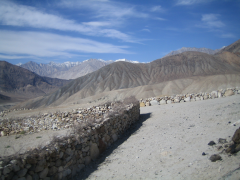< July 2006 > Su Mo Tu We Th Fr Sa 1 2 3 4 5 6 7 8 9 10 11 12 13 14 15 16 17 18 19 20 21 22 23 24 25 26 27 28 29 30 31
Tue, 18 Jul 2006:

## Cropped Rotation

The problem with taking photos in a moving car is that it is very hard to get the baseline of the photo on the horizontal. So eventually to get a decent pic, you need to rotate the photo. But after rotation, you need to crop it back to a square. All operations which take time and is relatively annoying. So, I wrote a gimp script.

```  r = math.sqrt(x*x + y*y)/2

# as calculated for top left corner
phi = math.radians(45 + angle)
dx = r * math.cos(phi) - r * math.cos(theta)
# -1 * because as the angle comes down, our y decreases
dy = -1 * (r * math.sin(phi) - r * math.cos(theta))
```

Basically, this python-fu script (rot-crop.py) does both operations together and gives you a cropped rectangular image.rotated 6 odd degrees

Script-Fu is esoteric stuff, but the python plugins can actually be read, understood and written by mere mortals like me.

--
My geometry teacher was sometimes acute, and sometimes obtuse, but always,
always, he was right.
[That's an interesting angle.  I wonder if there are any parallels?]

posted at: 02:22 | path: /hacks | permalink | Tags: , ,